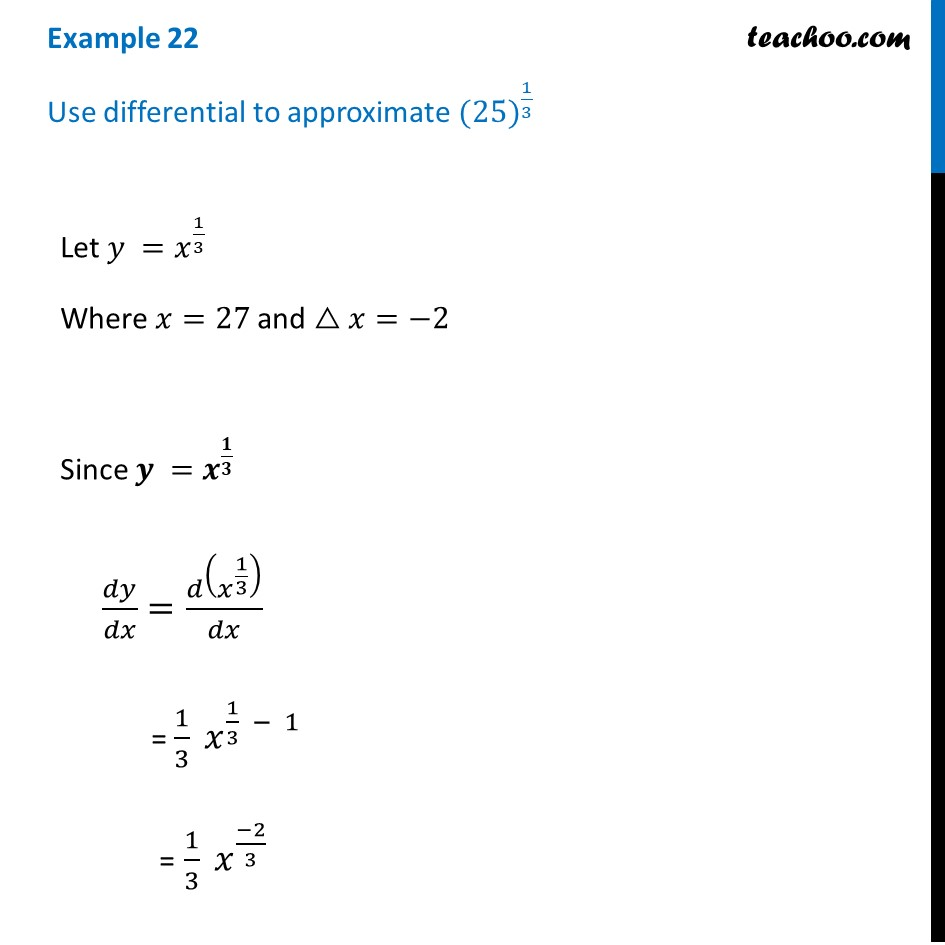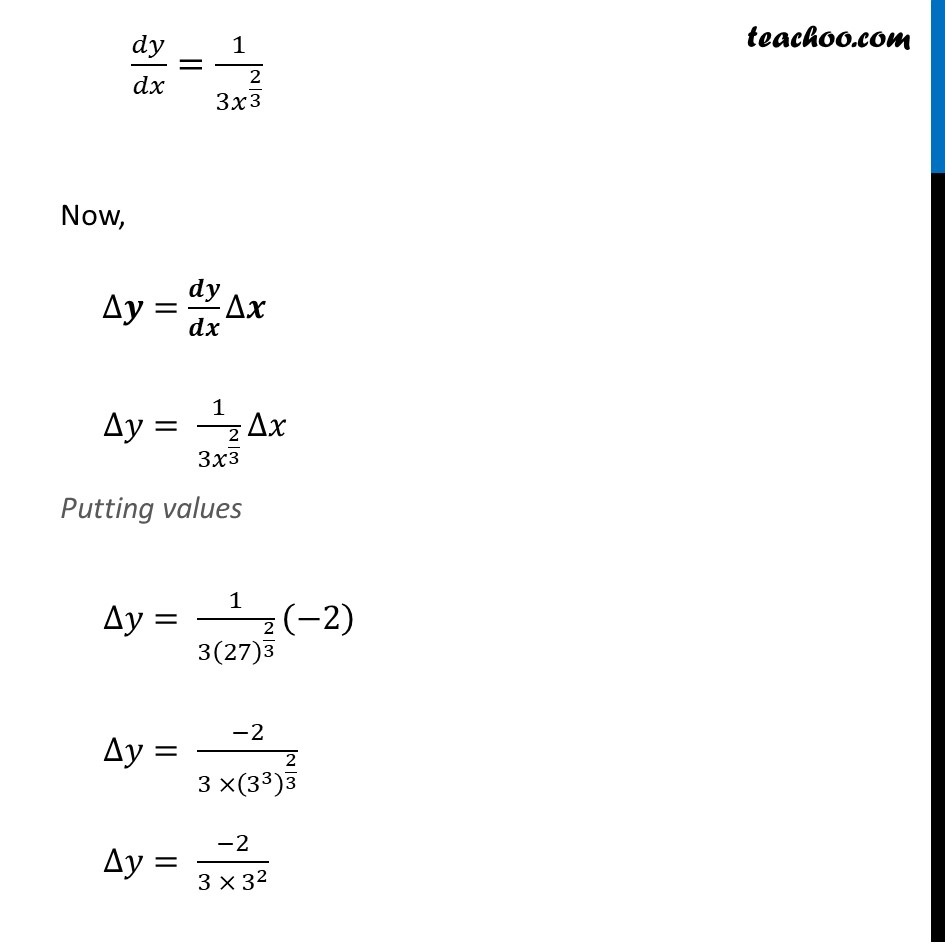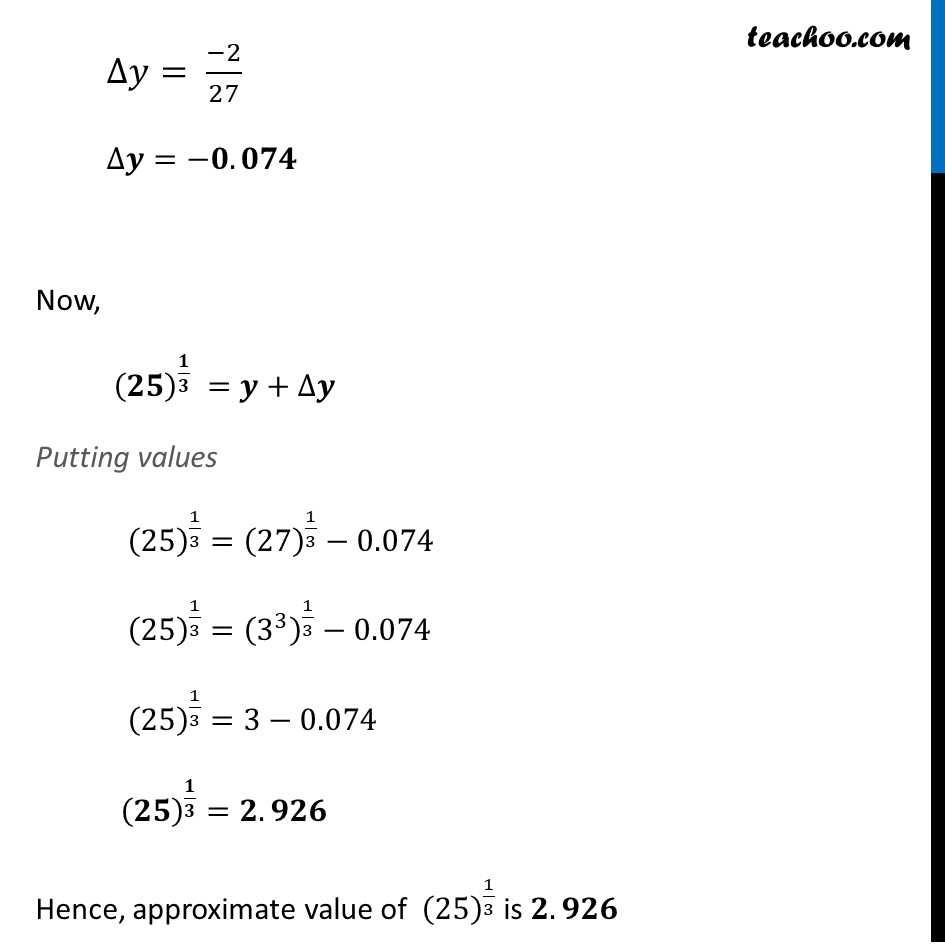Examples

Chapter 6 Class 12 Application of Derivatives
Serial order wiseLearn in your speed, with individual attention - Teachoo Maths 1-on-1 Class

### Transcript

Question 9 Use differential to approximate 〖(25)〗^(1/3)Let 𝑦 =𝑥^(1/3) Where 𝑥=27 and △𝑥=−2 Since 𝒚 =𝒙^(𝟏/𝟑) 𝑑𝑦/𝑑𝑥=𝑑(𝑥^(1/3) )/𝑑𝑥 = 1/3 𝑥^(1/3 − 1) = 1/3 𝑥^((−2)/3) 𝑑𝑦/𝑑𝑥=1/(3𝑥^(2/3) ) Now, ∆𝒚=𝒅𝒚/𝒅𝒙 ∆𝒙 ∆𝑦= 1/(3𝑥^(2/3) ) ∆𝑥 Putting values ∆𝑦= 1/(3(27)^(2/3) ) (−2) ∆𝑦= (−2)/(3 ×(3^3 )^(2/3) ) ∆𝑦= (−2)/(3 × 3^2 ) ∆𝑦= (−2)/27 ∆𝒚=−𝟎.𝟎𝟕𝟒 Now, (𝟐𝟓)^(𝟏/𝟑) =𝒚+∆𝒚 Putting values (25)^(1/3)=(27)^(1/3)−0.074 (25)^(1/3)=(3^3 )^(1/3)−0.074 (25)^(1/3)=3−0.074 (𝟐𝟓)^(𝟏/𝟑)=𝟐.𝟗𝟐𝟔 Hence, approximate value of (25)^(1/3) is 𝟐.𝟗𝟐𝟔